﻿ 一种微下击暴流的实时仿真及可视化方法 A Real-Time Simulation and Visualization of the Microburst

Computer Science and Application
Vol. 08  No. 10 ( 2018 ), Article ID: 27285 , 11 pages
10.12677/CSA.2018.810176

A Real-Time Simulation and Visualization of the Microburst

Jun Zhang1,2, Kaiwen Chen1

1School of Digital Media, Jiangnan University, Wuxi Jiangsu

2Jiangsu Key Laboratory of Media Design and Software Technology, Jiangnan University, Wuxi Jiangsu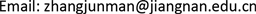Received: Oct. 4th, 2018; accepted: Oct. 19th, 2018; published: Oct. 26th, 2018ABSTRACT

The microburst is a special meteorological condition that affects the flight heavily. It threats to the aircraft seriously during takeoff and landing. A real-time visually simulation method of the microburst was presented to generate vivid 3D wind field for the flight simulation software or game. It may be used to improve pilots’ ability to deal with unexpected microburst situation. The presented method used a circle’s tangent model as a simplified mathematical approximation of the microburst. The simulated wind field was visualized by the common particle system overlapped a polygon trailing component. Experimental simulation scenes on the Unity engine show that the proposed method is easy to program and achieves real-time frame rate on a common PC.

Keywords:Microburst, Flight Simulation, Real-Time Simulation, Particle System, Visualization

1江南大学数字媒体学院，江苏 无锡

2江南大学江苏省媒体设计与软件技术重点实验室，江苏 无锡1. 引言

2. 微下击暴流仿真算法

2.1. 基于涡环的数学模型

Michael Ivan  最早根据气象观测数据提出基于涡环的微下击暴流仿真模型，其仿真结果与实测风场数据在视觉上具有较高的一致性，得到广泛应用。

$\left\{\begin{array}{l}{\left(x-{x}_{p}\right)}^{2}+{\left(y-{y}_{p}\right)}^{2}={R}^{2}\\ z={z}_{p}\end{array}$ (1)

${\psi }_{p}=\frac{\Gamma }{\text{2π}}\left({r}_{\mathrm{max}}+{r}_{\mathrm{min}}\right)F\left(k\right)$ (2)

$\left\{\begin{array}{l}{v}_{x}=\frac{{x}_{A}-{x}_{P}}{{r}_{p}}{v}_{r}\\ {v}_{y}=\frac{{y}_{A}-{y}_{P}}{{r}_{p}}{v}_{r}\\ {v}_{z}=-\frac{1}{{r}_{P}}\frac{\partial \left({\psi }_{I}+{\psi }_{P}\right)}{\partial r}\end{array}$ (3)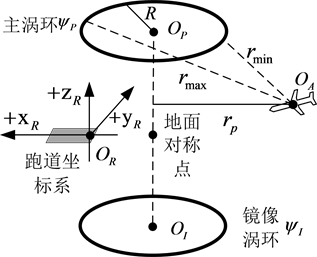Figure 1. The sketch illustration of the vortex ring model

Michael Ivan模型在计算上存在较大复杂度，对特殊位置(如主涡环自身和涡环中轴线等)存在奇异性问题，需要追加计算步骤。虽然后续研究者不断提出改进方案，但在计算速度上和程序复杂度上效果并不理想。

2.2. 本文圆切线模型

Michael Ivan模型采用涡环的主要原因是微下击暴流表现出典型的涡流现象，即风场存在一个旋转中心点，而方程(2)的切线方向与涡流现象吻合。方程(2)及其切线防线的计算复杂性是Michael Ivan模型复杂度的主要来源，本节提出使用圆切线的方式大幅降低模型复杂度。

Michael Ivan模型中计算涡环切线的过程，可以由图2中圆切线近似逼近，即风场在任意一点P的风矢量的方向与圆切线一致，大小与圆心距离调整：

$\left\{\begin{array}{l}{v}_{x}^{P}=\left(\frac{\left({y}_{p}-{y}_{B}\right)\varphi \left({d}_{B}\right)}{‖P-{O}_{B}‖}-\frac{\left({y}_{p}-{y}_{A}\right)\varphi \left({d}_{A}\right)}{‖P-{O}_{A}‖}\right)\stackrel{¯}{V}\\ {v}_{y}^{P}=\left(\frac{\left({x}_{P}-{x}_{A}\right)\varphi \left({d}_{A}\right)}{‖P-{O}_{A}‖}-\frac{\left({x}_{p}-{x}_{B}\right)\varphi \left({d}_{B}\right)}{‖P-{O}_{B}‖}\right)\stackrel{¯}{V}\end{array}$ (4)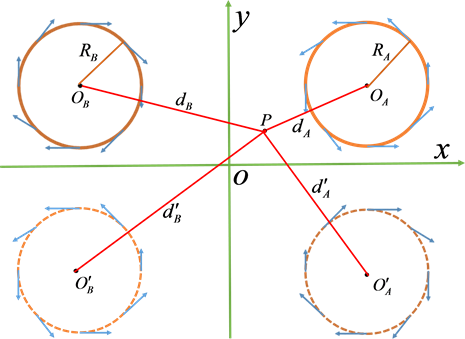Figure 2. The sketch illustration of the circle’s tangent model

3. 基于粒子系统的风场可视化技术

3.1. 粒子系统

$\left\{{\Theta }_{k}|{\Theta }_{k}=\left(\begin{array}{cccc}{x}_{k}& {y}_{k}& {z}_{k}& {t}_{k}\end{array}\right),k=1,2,\cdots ,N\right\}$ (5)

${\Theta }_{k}←\left(\begin{array}{c}{x}_{k}+{v}_{x}^{{\Theta }_{k}}\Delta t\\ {y}_{k}+{v}_{y}^{{\Theta }_{k}}\Delta t\\ {z}_{k}+{v}_{z}^{{\Theta }_{k}}\Delta t\end{array}\right)$ (6)

3.2. 拖尾技术

$\begin{array}{l}{T}_{0}={\Theta }_{k}\\ {T}_{k}={T}_{k-1}\end{array}$ (7)

4. 仿真实验结果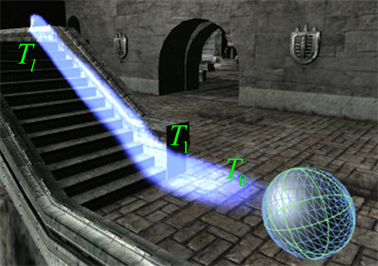Figure 3. Description of trailing algorithm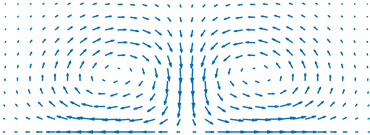(a) Michael Ivan模型仿真风场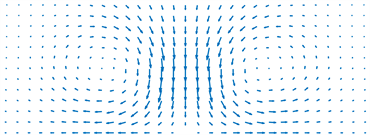(b) 本文方法仿真风场

Figure 4. Comparison of the simulated wind field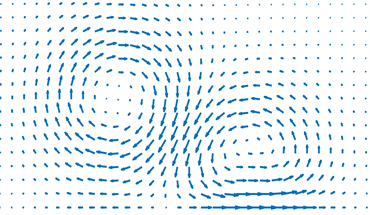(a) 参数设置一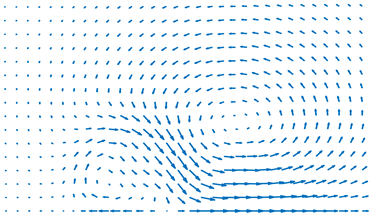(b) 参数设置二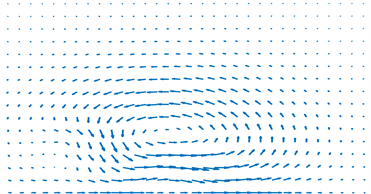(c) 参数设置三

Figure 5. Simulated wind field under different parameter settings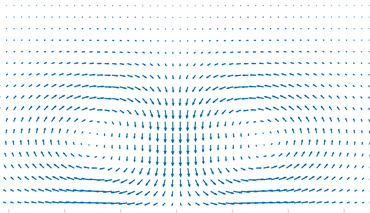Figure 6. Visualization results by traditional graph-based method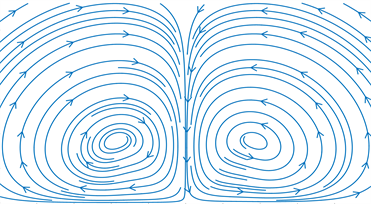Figure 7. Visualization results by traditional stream-line-based method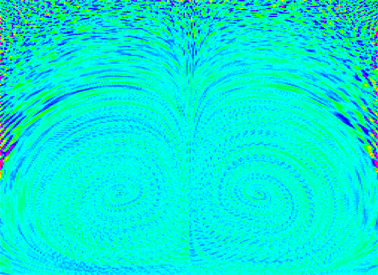Figure 8. Visualization results by traditional convolution-based methodFigure 9. Visualization results by our trail-based method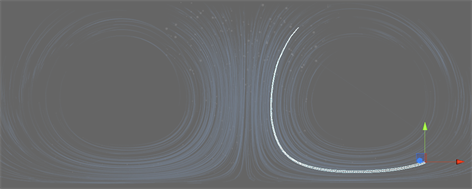Figure 10. A high lighted trail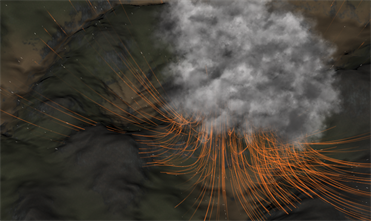Figure 11. Visualization results in a real 3D scene I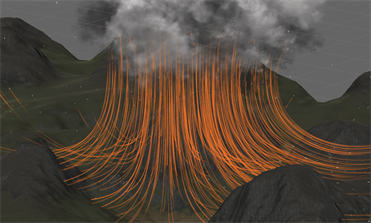Figure 12. Visualization results in a real 3D scene II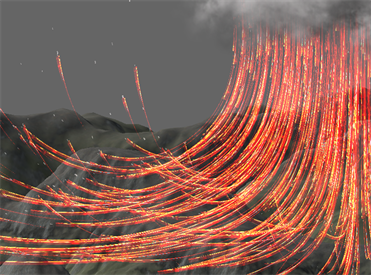Figure 13. Visualization results in a real 3D scene III

5. 结论Figure 14. A simulated and visualizated Microburst in a flight simulation scene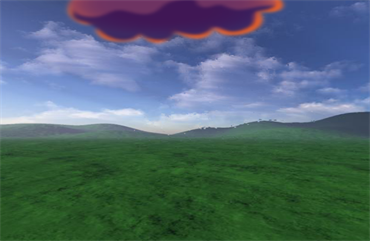Figure 15. Visualization results by the heat distortion trails

A Real-Time Simulation and Visualization of the Microburst[J]. 计算机科学与应用, 2018, 08(10): 1602-1612. https://doi.org/10.12677/CSA.2018.810176

1. 1. 谷润平, 崔锋. 飞机运行低空气象环境仿真建模分析[J]. 航空计算技术, 2015, 45(3): 1-7.

2. 2. Ivan, M. (1986) A Ring-Vortex Downburst Model for Flight Simulations. Journal of Aircraft, 23, 232-236. https://doi.org/10.2514/3.45294

3. 3. 韩雁飞, 刘夏, 李海, 等. 利用函数拟合的低空风切变三维建模与雷达回波仿真[J]. 信号处理, 2015, 31(9): 1180-1187.

4. 4. 陶杨, 韩维, 吴亮. 虚拟时变微下击暴流的数值仿真方法[J]. 应用力学学报, 2014, 31(6): 847-852.

5. 5. 畅刘, 滔李, 梅江涛, 等. 微下击暴流的三维建模与预警仿真研究[J]. 计算机仿真, 2011, 28(12): 47-52.

6. 6. Mason, M.S., Wood, G.S. and Fletcher, D.F. (2009) Numerical Simulation of Downburst Winds. Journal of Wind Engi-neering and Industrial Aerodynamics, 97, 523-539. https://doi.org/10.1016/j.jweia.2009.07.010

7. 7. 高振兴, 顾宏斌. 用于飞行实时仿真的微下击暴流建模研究[J]. 系统仿真学报, 2008, 20(23): 6524-6534.

8. 8. Gu, J., Tan, Z. and Qiu, X. (2015) Effects of Vertical Wind Shear on Inner-Core Thermodynamics of an Idealized Simulated Tropical Cyclone. Journal of the Atmospheric Sci-ences, 72, 511-530. https://doi.org/10.1175/JAS-D-14-0050.1

9. 9. Aboshosha, H., Bitsuamlak, G. and Damatty, A.E. (2015) Turbulence Characteri-zation of Downbursts Using LES. Journal of Wind Engineering and Industrial Aerodynamics, 136, 44-61. https://doi.org/10.1016/j.jweia.2014.10.020

10. 10. Cui, W.T., Liu, W.H. and Lei, X.Y. (2014) Modeling and Simulation of Micro-burst Wind Shear in the Flight Simulator. Proceedings of 2014 IEEE Chinese Guidance, Navigation and Control Conference, Yan Tai, 8-10 August 2014, 1095-1100. https://doi.org/10.1109/CGNCC.2014.7007358

11. 11. Orfa, L., Kantor, E. and Savory, E. (2012) Simulation of a Downburst-Producing Thunderstorm Using a Very High-Resolution Three-Dimensional Cloud Model. Journal of Wind Engineering and Industrial Aerodynamics, 104-106, 547-557. https://doi.org/10.1016/j.jweia.2012.02.020

12. 12. 李想, 乔木, 官巍, 等. 虚拟座舱技术在飞行模拟器中的应用[J]. 系统仿真学报, 2013, 25(增刊): 129-133.

13. 13. 郭林亮, 祝明红, 等. 一种低俗风动虚拟飞行试验装置的建模与仿真[J]. 空气动力学学报, 2017, 35(5): 708-717.

14. 14. Cabral, B. and Leedom, L. (1993) Imaging Vector Fields Using Line Integral Convolution. Proceedings of the 20th Annual Conference on Computer Graphics and In-teractive Techniques, Anaheim, CA, 2-6 August 1993, 263-270. https://doi.org/10.1145/166117.166151

15. 15. Forssell, L.K. and Cohen, S.D. (1995) Using Line Integral Convolution for Flow Visualization: Curvilinear Grids, Variable-Speed Animation, and Unsteady Flows. IEEE Transactions on Visualization and Computer Graphics, 1, 133-141. https://doi.org/10.1109/2945.468406

16. 16. Shen, H., Johnson, C.R. and Ma, K. (1996) Visualizing Vector Fields Using Line Inte-gral Convolution and Dye Advection. IEEE Symposium on Volume Visualization, San Franscisco, CA, 29-29 October 1996, 63-70.

17. 17. Van Wijk, J.J. (2002) Image Based Flow Visualization. ACM Transactions on Graphics, 21, 745-754. https://doi.org/10.1145/566654.566646

18. 18. Xu, H., Li, S., Zeng, L., et al. (2013) Feature-Based Adaptive Texture Visualization for Vector Field. Science China Information Sciences, 56, 1-14. https://doi.org/10.1007/s11432-011-4505-3

19. 19. 鲁大营, 朱登明, 王兆其. 基于纹理的多分辨率流场可视化算法[J]. 计算机研究与发展, 2015, 52(8): 1910-1920.

20. 20. 韩敏, 张海超, 边茂松, 等. 流线增强型线性积分卷积流场可视化[J]. 系统仿真学报, 2016, 28(12): 2933-2938.

21. NOTES

1请查看在线视频，其动态效果更佳直观。https://www.bilibili.com/video/av31249132/